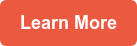As my fifth graders were nearing the end of our unit on volume, I went back to the Common Core State Standards to ensure that we had covered all required concepts thoroughly. I reread the fifth grade standard on measurement and data (5.MD.C.5b), where students apply two formulas (Volume = length x width x height and Volume = base x height) for volume of rectangular prisms to solve problems. I felt confident that my students understood and could efficiently use the first formula; however, I knew we needed to spend some more time with the second.

In our problem solving work, my students were intuitively solving using the second formula, but they were not aware that multiplying the area of the base by the height was a separate formula.

In my classroom, I never just give my students formulas. I have two reasons for this. First, formulas they memorize but don't understand are quickly forgotten. Second, if I want my students to think like mathematicians, then they need to discover formulas for themselves. Our experience building rectangular prisms had led naturally to the understanding of the formula V = l x w x h.

## Creating a Rich Math Task

I wanted the same thing to occur with V = b x h, but it was not happening. I looked online for resources to help me make a connection between the two formulas — but I could not find anything that inspired me. So I did what I do in these situations: I created a rich math task and ran it by my district math coach for feedback before using it in the classroom.

The format of the task was simple. I presented the "new formula" V = b x h to my students, telling them that my friend's fifth grade students on the East coast had developed a formula different from our formula of V = l x w x h. I asked them to critique the reasoning of the East coast students by determining if the new formula worked, and finding the similarities and differences between the formula they had been using and the new formula.

The lesson appeared to be so simple - the students only had to answer three questions - but I was not entirely sure how it would pan out in the classroom. But I was eager to try it. I was amazed that the three simple questions I asked led to powerful mathematical conversations. I was also excited because we had been working on critiquing the reasoning of others (Common Core Standard for Mathematical Practice #3), and this lesson was such a natural way to incorporate critiquing into the content they needed to learn.

Here are the three questions:

1. What do you think the variables in the Delaware students' formula represent?

2. Does their formula work? Explain your reasoning.

3. How is it similar and different to your formula?

## Productive Conversation

I could not have asked for richer, more productive partner and whole group student conversations. (See examples of student work below.) I really wish I had videotaped the discussion! The students' ideas and connections blew me away. Students went beyond just telling the class that the new formula worked. They connected the base in the new formula with the concept of length x width in their formula, and were able to explain why these two ideas were related using precise math vocabulary (for example, "base is the bottom layer of the rectangular prism," or "base is the area of the base of the rectangular prism").

They even corrected me by pointing out I should not have used the word "it" in one of the questions. They reminded me that mathematicians are specific and do not use the word "it." They also discussed the pros and cons of each formula, and when they might be useful in certain situations.

One of the coolest connections happened when one of my boys stated that the East coast students using the new formula "are still doing the same amount of work as us." This spurred a conversation about how the new formula was written more simply, but actually required the same steps as the formula my students had developed.

Finally, we ended the class with a discussion about when they might see the new formula again in the future. We talked about how V = b x h could be used to calculate the volume of a triangular prism and a cylinder. (This made the middle school teacher in me happy!)

Critiquing the reasoning of others is such a powerful mathematical practice. I was amazed how the students in my classroom used this task to learn more than just the content. I truly felt that my little mathematicians were engaging in real world skills -- looking at the work of another person and deciding whether it works and how it connects.

Topics: Lesson Planning, Math#### Written by Alicia Farmer

Alicia Farmer is a fifth grade math teacher in Anacortes, WA, and this is her tenth year of teaching. Alicia has taught middle school math, science, history, self-contained sixth grade, and most recently fifth grade math. She has her National Board certification in the area of Early Adolescent Mathematics.

## Comments

Sign up for the Teaching Channel newsletter to get the latest articles, videos, and resources delivered to your inbox every Saturday morning.

### Search Blogs

• Content Title

Description

• ### Articles by Topic

WANT TO PARTNER WITH US?

We're always looking for new TCHERS' VOICE bloggers! If you're interested in writing an article, please get in touch with us.